# Python | Pandas dataframe.all()

Python is a great language for doing data analysis, primarily because of the fantastic ecosystem of data-centric python packages. Pandas is one of those packages and makes importing and analyzing data much easier.

`DataFrame.all()` method checks whether all elements are True, potentially over an axis. It returns True if all elements within a series or along a Dataframe axis are non-zero, not-empty or not-False.

Syntax: DataFrame.all(axis=0, bool_only=None, skipna=True, level=None, **kwargs)

Parameters:
axis : {0 or ‘index’, 1 or ‘columns’, None}, default 0
Indicate which axis or axes should be reduced.
0 / ‘index’ : reduce the index, return a Series whose index is the original column labels.
1 / ‘columns’ : reduce the columns, return a Series whose index is the original index.
None : reduce all axes, return a scalar.

skipna : Exclude NA/null values. If an entire row/column is NA, the result will be NA.
level : If the axis is a MultiIndex (hierarchical), count along a particular level, collapsing into a Series.
bool_only : Include only boolean columns. If None, will attempt to use everything, then use only boolean data. Not implemented for Series.
**kwargs : Additional keywords have no effect but might be accepted for compatibility with NumPy.

Returns: all : Series or DataFrame (if level specified)

Note: Nan value will be treated as non-empty value and hence it will be evaluated as True.

Example #1: Suffix `_col `in each columns in the dataframe.

 `# importing pandas as pd ` `import` `pandas as pd ` ` `  `# Making data frame from the csv file ` `df ``=` `pd.read_csv(``"nba.csv"``) ` ` `  `# Printing the first 10 rows of the ` `# data frame for visualization ` `df[:``10``] `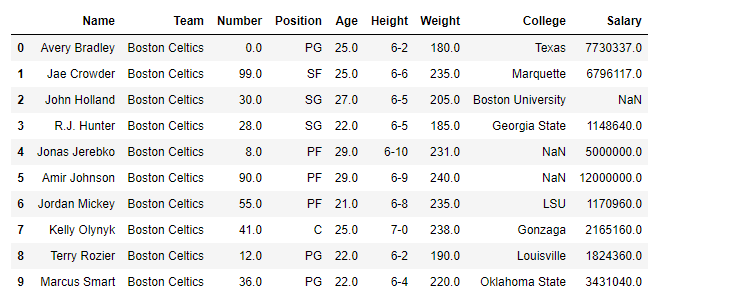`# checking for 'Name' column ` `df.Name.``all``() `

Output: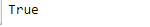Example #2: Evaluate for column-wise behaviour

`dataframe.all()` Default behaviour checks if column-wise values all return True.

 `# Checking for all the columns in the dataframe ` `df.``all``() `

Output: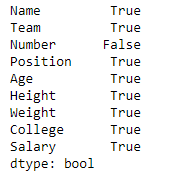Example #3: Check for row-wise elements

Specify axis=’columns’ to check if row-wise values all return True. if all values in any specific row evaluate to true then the overall row will be evaluated as true.

 `# importing pandas as pd ` `import` `pandas as pd ` ` `  `# Making data frame from the csv file ` `df ``=` `pd.read_csv(``"nba.csv"``) ` ` `  `# Checking across the row ` `df.``all``(axis ``=``'columns'``) `

Output: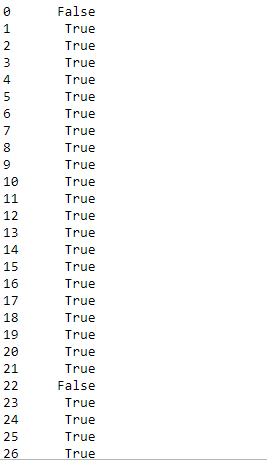`all() `evaluates all value across all of the rows in the dataframe and outputs a boolean value for each row.

Example #4: Check for all values in the dataframe

Specify, axis=None for whether every value is True in the dataframe.

 `# importing pandas as pd ` `import` `pandas as pd ` ` `  `# Making data frame from the csv file ` `df ``=` `pd.read_csv(``"nba.csv"``) ` ` `  `# Checking across the row ` `df.``all``(axis ``=` `None``) `

Output: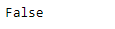My Personal Notes arrow_drop_upCheck out this Author's contributed articles.

If you like GeeksforGeeks and would like to contribute, you can also write an article using contribute.geeksforgeeks.org or mail your article to contribute@geeksforgeeks.org. See your article appearing on the GeeksforGeeks main page and help other Geeks.

Please Improve this article if you find anything incorrect by clicking on the "Improve Article" button below.

Article Tags :

Be the First to upvote.

Please write to us at contribute@geeksforgeeks.org to report any issue with the above content.